### Home > PC > Chapter 8 > Lesson 8.2.1 > Problem8-71

8-71.
1. Find the following sums. You can use your SUM program or the sum sequence commands from your calculator. Homework Help ✎

1.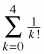2.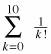3. What is the value of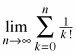?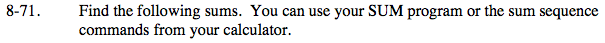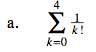$=\frac{1}{0!}+\frac{1}{1!}+\frac{1}{2!}+\frac{1}{3!}+\frac{1}{4!} =1+1+\frac{1}{2}+\frac{1}{6}+\frac{1}{24} =2.708333$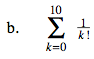2.71828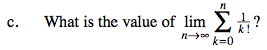e